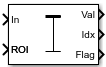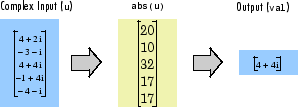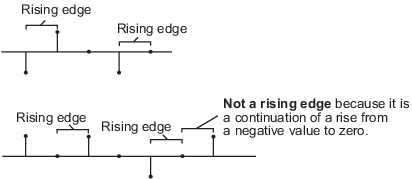Documentation

# 2-D Maximum

Find maximum values in input or sequence of inputs

## Library

Statistics

`visionstatistics`## Description

The 2-D Maximum block identifies the value and/or position of the smallest element in each row or column of the input, or along a specified dimension of the input. The 2-D Maximum block can also track the maximum values in a sequence of inputs over a period of time.

The 2-D Maximum block supports real and complex floating-point, fixed-point, and Boolean inputs. Real fixed-point inputs can be either signed or unsigned, while complex fixed-point inputs must be signed. The output data type of the maximum values match the data type of the input. The block outputs `double` index values, when the input is `double`, and `uint32` otherwise.

### Port Descriptions

PortInput/OutputSupported Data Types

Input

Scalar, vector or matrix of intensity values

• Double-precision floating point

• Single-precision floating point

• Fixed point (signed and unsigned)

• Boolean

• 8-, 16-, and 32-bit signed integers

• 8-, 16-, and 32-bit unsigned integers

Rst

Scalar value

• Double-precision floating point

• Single-precision floating point

• Boolean

• 8-, 16-, and 32-bit signed integers

• 8-, 16-, and 32-bit unsigned integers

Val

Maximum value output based on the Value Mode

• Double-precision floating point

• Single-precision floating point

• Fixed point (signed and unsigned)

• Boolean

• 8-, 16-, and 32-bit signed integers

• 8-, 16-, and 32-bit unsigned integers

Idx

One-based output location of the maximum value based on the Index Mode

• Double-precision floating point

• 32-bit unsigned integers

### Value Mode

When you set the Mode parameter to `Value`, the block computes the maximum value in each row or column of the input, along vectors of a specified dimension of the input, or of the entire input at each sample time, and outputs the array y. Each element in y is the maximum value in the corresponding column, row, vector, or entire input. The output y depends on the setting of the Find the maximum value over parameter. For example, consider a 3-dimensional input signal of size M-by-N-by-P:

• `Each row` — The output at each sample time consists of an M-by-1-by-P array, where each element contains the maximum value of each vector over the second dimension of the input. For an M-by-N input matrix, the block outputs an M-by-1 column vector at each sample time.

• `Each column` — The output at each sample time consists of a 1-by-N-by-P array, where each element contains the maximum value of each vector over the first dimension of the input. For an M-by-N input matrix, the block outputs a 1-by-N row vector at each sample time.

In this mode, the block treats length-M unoriented vector inputs as M-by-1 column vectors.

• `Entire input` — The output at each sample time is a scalar that contains the maximum value in the M-by-N-by-P input matrix.

• `Specified dimension` — The output at each sample time depends on Dimension. When you set Dimension to `1`, the block output is the same as when you select ```Each column```. When you set Dimension to `2`, the block output is the same as when you select `Each row`. When you set Dimension to `3`, the block outputs an M-by-N matrix containing the maximum value of each vector over the third dimension of the input, at each sample time.

For complex inputs, the block selects the value in each row or column of the input, along vectors of a specified dimension of the input, or of the entire input that has the maximum magnitude squared as shown below. For complex value $u=a+bi$, the magnitude squared is ${a}^{2}+{b}^{2}$.### Index Mode

When you set the Mode parameter to `Index`, the block computes the maximum value in each row or column of the input, along vectors of a specified dimension of the input, or of the entire input, and outputs the index array I. Each element in I is an integer indexing the maximum value in the corresponding column, row, vector, or entire input. The output I depends on the setting of the Find the maximum value over parameter. For example, consider a 3-dimensional input signal of size M-by-N-by-P:

• `Each row` — The output at each sample time consists of an M-by-1-by-P array, where each element contains the index of the maximum value of each vector over the second dimension of the input. For an input that is an M-by-N matrix, the output at each sample time is an M-by-1 column vector.

• `Each column` — The output at each sample time consists of a 1-by-N-by-P array, where each element contains the index of the maximum value of each vector over the first dimension of the input. For an input that is an M-by-N matrix, the output at each sample time is a 1-by-N row vector.

In this mode, the block treats length-M unoriented vector inputs as M-by-1 column vectors.

• `Entire input` — The output at each sample time is a 1-by-3 vector that contains the location of the maximum value in the M-by-N-by-P input matrix. For an input that is an M-by-N matrix, the output will be a 1-by-2 vector of one-based [x y] location coordinates for the maximum value.

• `Specified dimension` — The output at each sample time depends on Dimension. If Dimension is set to `1`, the output is the same as when you select ```Each column```. If Dimension is set to `2`, the output is the same as when you select `Each row`. If Dimension is set to `3`, the output at each sample time is an M-by-N matrix containing the indices of the maximum values of each vector over the third dimension of the input.

When a maximum value occurs more than once, the computed index corresponds to the first occurrence. For example, when the input is the column vector `[3 2 1 2 3]'`, the computed one-based index of the maximum value is `1` rather than `5` when ```Each column``` is selected.

When inputs to the block are double-precision values, the index values are double-precision values. Otherwise, the index values are 32-bit unsigned integer values.

### Value and Index Mode

When you set the Mode parameter to ```Value and Index```, the block outputs both the maxima and the indices.

### Running Mode

When you set the Mode parameter to `Running`, the block tracks the maximum value of each channel in a time sequence of M-by-N inputs. In this mode, the block treats each element as a channel.

### Resetting the Running Maximum

The block resets the running maximum whenever a reset event is detected at the optional Rst port. The reset sample time must be a positive integer multiple of the input sample time.

You specify the reset event in the Reset port menu:

• `None` — Disables the Rst port.

• `Rising edge` — Triggers a reset operation when the Rst input does one of the following:

• Rises from a negative value to a positive value or zero

• Rises from zero to a positive value, where the rise is not a continuation of a rise from a negative value to zero (see the following figure)• `Falling edge` — Triggers a reset operation when the `Rst` input does one of the following:

• Falls from a positive value to a negative value or zero

• Falls from zero to a negative value, where the fall is not a continuation of a fall from a positive value to zero (see the following figure)• `Either edge` — Triggers a reset operation when the `Rst` input is a ```Rising edge``` or `Falling edge` (as described above)

• `Non-zero sample` — Triggers a reset operation at each sample time that the `Rst` input is not zero

### Note

When running simulations in the Simulink® `MultiTasking` mode, reset signals have a one-sample latency. Therefore, when the block detects a reset event, there is a one-sample delay at the reset port rate before the block applies the reset.

### ROI Processing

To calculate the statistical value within a particular region of interest (ROI) of the input, select the Enable ROI processing check box. This applies to any mode other than running mode and when you set the Find the maximum value over parameter to `Entire input` and you select the Enable ROI processing check box. ROI processing applies only for 2-D inputs.

You can specify `Rectangles`, `Lines`, `Label matrix`, or ```Binary mask``` ROI type.

Use the `Binary mask` to specify which pixels to highlight or select.

Use the `Label matrix` to label regions. Pixels set to `0` represent the background. Pixels set to `1` represent the first object, pixels set to `2`, represent the second object, and so on. Use the Label Numbers port to specify the objects in the label matrix for which the block calculates statistics. The input to this port must be a vector of scalar values that correspond to the labeled regions in the label matrix.

For rectangular ROIs, use the ROI portion to process parameter to specify whether to calculate the statistical value for the entire ROI or just the ROI perimeter. For more information about the format of the input to the ROI port when you set the ROI to a rectangle or a line, see the Draw Shapes block reference page.

#### ROI Output Statistics

Output = Individual statistics for each ROI

Flag Port OutputDescription
0ROI is completely outside the input image.
1ROI is completely or partially inside the input image.

Output = Single statistic for all ROIs

Flag Port OutputDescription
0All ROIs are completely outside the input image.
1At least one ROI is completely or partially inside the input image.

If the ROI is partially outside the image, the block only computes the statistical values for the portion of the ROI that is within the image.

Output = Individual statistics for each ROI

Flag Port OutputDescription
0Label number is not in the label matrix.
1Label number is in the label matrix.

Output = Single statistic for all ROIs

Flag Port OutputDescription
0None of the label numbers are in the label matrix.
1At least one of the label numbers is in the label matrix.

### Fixed-Point Data Types

The parameters on the Data Types pane of the block dialog are only used for complex fixed-point inputs. The sum of the squares of the real and imaginary parts of such an input are formed before a comparison is made, as described in Value Mode. The results of the squares of the real and imaginary parts are placed into the product output data type. The result of the sum of the squares is placed into the accumulator data type. These parameters are ignored for other types of inputs.

## Parameters

Mode

Specify the block's mode of operation:

• `Value and Index` — Output both the value and the index location.

• `Value` — Output the maximum value of each input matrix. For more information, see Value Mode.

• `Index`— Output the one-based index location of the maximum value. For more information, see Index Mode.

• `Running` — Track the maximum value of the input sequence over time. For more information, see Running Mode.

For the `Value`, `Index`, and `Value and Index` modes, the 2-D Maximum block produces identical results as the MATLAB® `max` function when it is called as `[y I] = max(u,[],D)`, where `u` and `y` are the input and output, respectively, D is the dimension, and I is the index.

Find the maximum value over

Specify whether the block should find the maximum of the entire input each row, each column, or dimensions specified by the Dimension parameter.

Reset port

Specify the reset event that causes the block to reset the running maximum. The rate of the reset signal must be a positive integer multiple of the rate of the data signal input. This parameter appears only when you set the Mode parameter to `Running`. For information about the possible values of this parameter, see Resetting the Running Maximum.

Dimension

Specify the dimension (one-based value) of the input signal, over which the maximum is computed. The value of this parameter cannot exceed the number of dimensions in the input signal. This parameter applies only when you set the Find the maximum value over parameter to `Specified dimension`.

Enable ROI processing

Select this check box to calculate the statistical value within a particular region of each image. This parameter applies only when you set the Find the maximum value over parameter to `Entire input`, and the block is not in running mode.

ROI type

Specify the type of ROI you want to use. Your choices are `Rectangles`, `Lines`, `Label matrix`, or ```Binary mask```.

When you set this parameter to `Rectangles` or `Lines`, the Output flag indicating if ROI is within image bounds check box appears in the dialog box. If you select this check box, the Flag port appears on the block.

When you set this parameter to `Label matrix`, the Label and Label Numbers ports appear on the block and the Output flag indicating if input label numbers are valid check box appears in the dialog box. If you select this check box, the Flag port appears on the block.

See ROI Output Statistics for details.

ROI portion to process

Specify whether you want to calculate the statistical value for the entire ROI or just the ROI perimeter. This parameter applies only when you set the ROI type parameter to `Rectangles`.

Output

Specify the block output. The block can output a vector of separate statistical values for each ROI or a scalar value that represents the statistical value for all the specified ROIs. This parameter does not apply when you set the ROI type parameter, to `Binary mask`.

Output flag indicating if ROI is within image bounds

When you select this check box, the Flag port appears on the block. This check box applies only when you set the ROI type parameter to `Rectangles` or `Lines`. For a description of the Flag port output, see the tables in ROI Processing.

Output flag indicating if label numbers are valid

When you select this check box, the Flag port appears on the block. This check box applies only when you set the ROI type parameter to `Label matrix`. For a description of the Flag port output, see the tables in ROI Processing.

### Note

The parameters on the Data Types pane are only used for complex fixed-point inputs. The sum of the squares of the real and imaginary parts of such an input are formed before a comparison is made, as described in Value Mode. The results of the squares of the real and imaginary parts are placed into the product output data type. The result of the sum of the squares is placed into the accumulator data type. These parameters are ignored for other types of inputs.

Rounding mode

Select the Rounding Modes for fixed-point operations.

Saturate on integer overflow

Select the overflow mode for fixed-point operations. See Precision and Range.

Product output data type

Specify the product output data type. See Fixed-Point Data Types and Multiplication Data Types for illustrations depicting the use of the product output data type in this block. You can set it to:

• A rule that inherits a data type, for example, ```Inherit: Same as input```

• An expression that evaluates to a valid data type, for example, `fixdt([],16,0)`

Click the buttonto display the Data Type Assistant, which helps you set the Product output data type parameter.

See Specify Data Types Using Data Type Assistant (Simulink) for more information.

Accumulator data type

Specify the accumulator data type. See Fixed-Point Data Types for illustrations depicting the use of the accumulator data type in this block. You can set this parameter to:

• A rule that inherits a data type, for example, ```Inherit: Same as product output```

• An expression that evaluates to a valid data type, for example, `fixdt([],16,0)`

Click the buttonto display the Data Type Assistant, which helps you set the Accumulator data type parameter.

See Specify Data Types Using Data Type Assistant (Simulink) for more information.

Lock data type settings against changes by the fixed-point tools

Select this parameter to prevent the fixed-point tools from overriding the data types you specify on the block mask.

## ExamplesThe ex_vision_2dmaximum example finds the maximum value within two ROIs. The model outputs the maximum values and their one-based [x y] coordinate locations.

## See Also

 2-D Mean Computer Vision System Toolbox 2-D Minimum Computer Vision System Toolbox MinMax Simulink `max` MATLAB

Download ebook